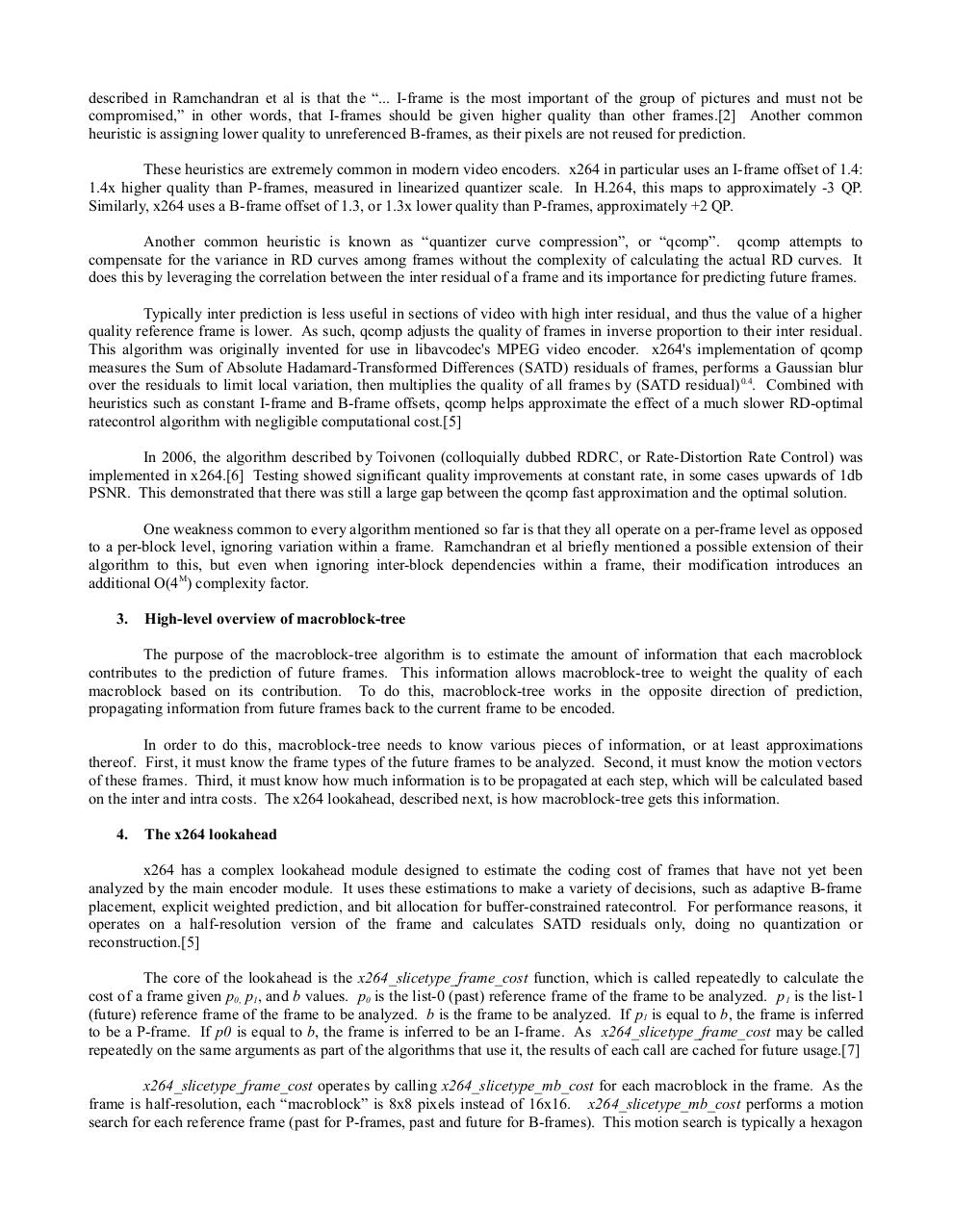# MBtree paper.pdfPage 1 2 3 4 5 6 7 8 9 10 11 12

#### Text preview

described in Ramchandran et al is that the “... I-frame is the most important of the group of pictures and must not be
compromised,” in other words, that I-frames should be given higher quality than other frames. Another common
heuristic is assigning lower quality to unreferenced B-frames, as their pixels are not reused for prediction.
These heuristics are extremely common in modern video encoders. x264 in particular uses an I-frame offset of 1.4:
1.4x higher quality than P-frames, measured in linearized quantizer scale. In H.264, this maps to approximately -3 QP.
Similarly, x264 uses a B-frame offset of 1.3, or 1.3x lower quality than P-frames, approximately +2 QP.
Another common heuristic is known as “quantizer curve compression”, or “qcomp”. qcomp attempts to
compensate for the variance in RD curves among frames without the complexity of calculating the actual RD curves. It
does this by leveraging the correlation between the inter residual of a frame and its importance for predicting future frames.
Typically inter prediction is less useful in sections of video with high inter residual, and thus the value of a higher
quality reference frame is lower. As such, qcomp adjusts the quality of frames in inverse proportion to their inter residual.
This algorithm was originally invented for use in libavcodec's MPEG video encoder. x264's implementation of qcomp
measures the Sum of Absolute Hadamard-Transformed Differences (SATD) residuals of frames, performs a Gaussian blur
over the residuals to limit local variation, then multiplies the quality of all frames by (SATD residual) 0.4. Combined with
heuristics such as constant I-frame and B-frame offsets, qcomp helps approximate the effect of a much slower RD-optimal
ratecontrol algorithm with negligible computational cost.
In 2006, the algorithm described by Toivonen (colloquially dubbed RDRC, or Rate-Distortion Rate Control) was
implemented in x264. Testing showed significant quality improvements at constant rate, in some cases upwards of 1db
PSNR. This demonstrated that there was still a large gap between the qcomp fast approximation and the optimal solution.
One weakness common to every algorithm mentioned so far is that they all operate on a per-frame level as opposed
to a per-block level, ignoring variation within a frame. Ramchandran et al briefly mentioned a possible extension of their
algorithm to this, but even when ignoring inter-block dependencies within a frame, their modification introduces an
3.

High-level overview of macroblock-tree

The purpose of the macroblock-tree algorithm is to estimate the amount of information that each macroblock
contributes to the prediction of future frames. This information allows macroblock-tree to weight the quality of each
macroblock based on its contribution. To do this, macroblock-tree works in the opposite direction of prediction,
propagating information from future frames back to the current frame to be encoded.
In order to do this, macroblock-tree needs to know various pieces of information, or at least approximations
thereof. First, it must know the frame types of the future frames to be analyzed. Second, it must know the motion vectors
of these frames. Third, it must know how much information is to be propagated at each step, which will be calculated based
on the inter and intra costs. The x264 lookahead, described next, is how macroblock-tree gets this information.
4.

x264 has a complex lookahead module designed to estimate the coding cost of frames that have not yet been
analyzed by the main encoder module. It uses these estimations to make a variety of decisions, such as adaptive B-frame
placement, explicit weighted prediction, and bit allocation for buffer-constrained ratecontrol. For performance reasons, it
operates on a half-resolution version of the frame and calculates SATD residuals only, doing no quantization or
reconstruction.
The core of the lookahead is the x264_slicetype_frame_cost function, which is called repeatedly to calculate the
cost of a frame given p0, p1, and b values. p0 is the list-0 (past) reference frame of the frame to be analyzed. p1 is the list-1
(future) reference frame of the frame to be analyzed. b is the frame to be analyzed. If p1 is equal to b, the frame is inferred
to be a P-frame. If p0 is equal to b, the frame is inferred to be an I-frame. As x264_slicetype_frame_cost may be called
repeatedly on the same arguments as part of the algorithms that use it, the results of each call are cached for future usage.
x264_slicetype_frame_cost operates by calling x264_slicetype_mb_cost for each macroblock in the frame. As the
frame is half-resolution, each “macroblock” is 8x8 pixels instead of 16x16. x264_slicetype_mb_cost performs a motion
search for each reference frame (past for P-frames, past and future for B-frames). This motion search is typically a hexagon